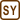## Heating of a Nail When Hammered

Steel nail with a mass of 50 g is hammered into a board by 20 hammer blows. The hammer mass is 0.5 kg and the impact velocity is 12 ms−1.

We assume that the hammer is completely brought to rest after each blow.

What is the nail temperature increase assuming that the nail absorbs 70 % of energy converted at each hammer blow?

• #### Hint

What kind of the hammer energy transforms when the hammer strikes the nail and what form of energy does it transform into?

• #### Analysis

When the hammer strikes the nail, the kinetic energy of the hammer converts into the internal energy. The nail, however, absorbs only a given part of this energy (70 %) which increases the nail temperature.

First we will calculate the total energy released, then the part that the nail actually absorbs, and finally we will calculate the resulting temperature.

• #### Numerical values

 m = 50 g = 0.05 kg the mass of the nail n = 20 the number of hammer blows mh = 0.5 kg the mass of the hammer v = 12 m s−1 the hammer impact velocity η = 70 % = 0.70 the proportion of the energy absorbed by a nail ΔT = ? the change of the nail temperature

From the Handbook of Chemistry and Physics:

 c = 482 J kg−1 K−1 the specific heat capacity of steel
• #### Solution

First, we calculate the total energy E1 released when the hammer strikes the nail. As the hammer is brought to rest after each blow, the energy released is equal to the total kinetic energy of the hammer right before the impact.

Therefore we can write:

$E_{1} = nE_{k} = \frac{1}{2}nm_{h}v^{2},$

where n is the number of hammer blows, mh the mass of the hammer and v its impact velocity.

However, the nail absorbs only a portion of the heat generated this way (namely η = 70 % = 0.7):

$E_{2} = \eta E_{1} = \frac{\eta nm_{h}v^{2}}{2}\, .$

This energy is manifested by increasing the nail temperature. Therefore we can write:

$E_{2} = mc\mathrm{\Delta} T$ $\mathrm{\Delta} T = \frac{E_{2}}{mc} = \frac{\eta nm_{h}v^{2}}{2mc},$

where m is the mass of the nail and c is the specific heat capacity of steel.

• #### Numerical insertion

$\mathrm{\Delta} T = \frac{\eta nm_{h}v^{2}}{2mc} = \frac{0.70\cdot{20}\cdot 0.5\cdot{12^{2}}}{2\cdot{ 0.05}\cdot{482}}\, \mathrm{K}$ $\mathrm{\Delta} T \dot= 21\, \mathrm{K}\, \Rightarrow\, \mathrm{\Delta}t \dot= 21\, \mathrm{^{\circ}C}$